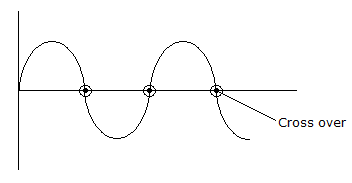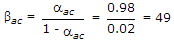Electronics and Communication Engineering - Electronic Devices and Circuits

26.

Crossover distortion behaviour is characteristic of

 A. class A O/P stage B. class B O/P stage C. class AB output stage D. common pulse O/P state

Explanation:

It is a characteristics of class B output stage as the amplifier is biased in cut-off region.

In class B amplifier, two transistor are operated in such a way that one is amplify the half cycle and second is amplify -ve half cycle.27.

If aac for transistor is 0.98 then βac is equal to

 A. 51 B. 49 C. 47 D. 45

Explanation:.

28.

Assertion (A): The conductivity of p type semiconductor is higher than that of intrinsic semiconductor.

Reason (R): The addition of donor impurity creates additional energy levels below conduction band.

 A. Both A and R are true and R is correct explanation of A B. Both A and R are true but R is not a correct explanation of A C. A is true but R is false D. A is false but R is true

Explanation:

A refers to type semiconductor while R refers to n type semiconductor. Both A and R are correct but independent.

29.

In an n-p-n transistor biased for operation in forward active region

 A. emitter is positive with respect to base B. collector is positive with respect to base C. base is positive with respect to emitter and collector is positive with respect to base D. none of the above

Explanation:

In forward active mode emitter base junction is forward biased and base collector junction is reverse biased.

30.

An increase in temperature increases the width of depletion layer.

 A. True B. False